• info@gomaths.co.za
• +27 (010) 312 6485

# Classifying Solids

Solid figures are classified into prisms, pyramids, cylinders, spheres and so on. A prism is a solid that has two congruent bases which are parallel faces. The other faces of the prism are in the shape of rectangles. They are called lateral faces. A prism is named after the shape of its base. The following diagrams show a triangular prism and a rectangular prism. A right prism is a prism that has its bases perpendicular to its lateral surfaces. A Rectangular prism is a prism that has rectangles as its bases. A shoe box, a soap box, a book, etc., are examples of rectangular prism.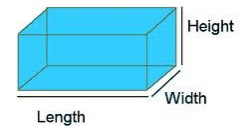A Cube is a special case of a rectangular prism where all faces and bases are congruent squares. A dice, ice cubes, Rubik’s cube and so on are real world examples of cube shape.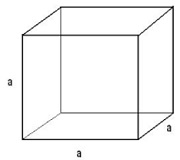A triangular prism is a prism that has triangles as its bases.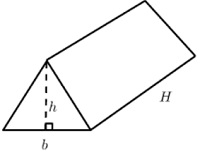Prisms are named after the shape of their bases. A pentagonal prism has pentagons as its bases, a hexagonal prism has hexagons as its bases and so on.

## Cylinder

A Cylinder is a closed solid figure having two parallel circular bases connected by a rectangular curved face.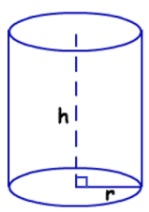A Sphere is solid round figure where every point on its surface is equidistant from its centre.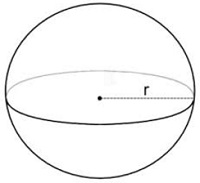A Cone is a closed solid figure whose circular base tapers into a point called apex.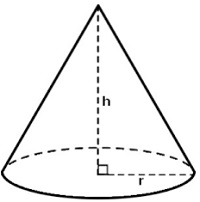## Pyramids

A pyramid is a solid figure that has a base, which can be any polygon, and three or more triangular faces that meet at a point called the apex. These triangular sides are called the lateral faces.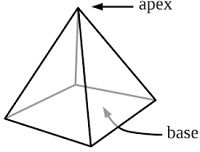Use the diagram to identify the name of the following shape.### Solution

Step 1: The given figure is a prism whose bases are congruent rectangles. Step 2: The given figure is a rectangular prism.
Use the diagram to identify the name of the following shape.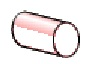### Solution

Step 1: The given figure is a solid figure whose bases are congruent circles. Step 2: The given figure is a cylinder
Back to: 3d Figures and Volumes > Chapter 1: Welcome. (Marc Twain)